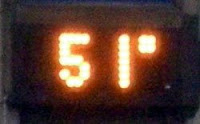## Wednesday, December 3, 2008

### 51

51 = 3 x 17.

51 is the smallest number that can be written with all the digits from 1 to 5 (without repetition) as a sum of prime numbers: 2 + 3 + 5 + 41 = 51. Note that the highest digit (5) and the lowest digit (1) are the digits of 51.

51 is a pentagonal number, a number of the form n(3n - 1)/2, and a centered pentagonal number (one of the few numbers to be both).

51 is 110011 in base 2 (binary) and 33 in base 16.

51 is the sixth Motzkin number.Area 51 is a nickname for a secret military base located in the southern portion of Nevada in the western United States.

Source: Prime Curios!